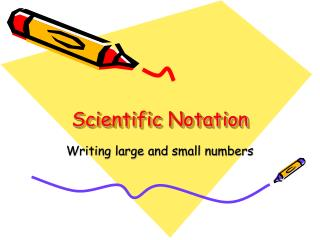DownloadDownload PresentationScientific Notation

# Scientific Notation

Download Presentation## Scientific Notation

- - - - - - - - - - - - - - - - - - - - - - - - - - - E N D - - - - - - - - - - - - - - - - - - - - - - - - - - -
##### Presentation Transcript

1. Scientific Notation Writing large and small numbers

2. It’s easy to make mistakes when computing with small numbers such as 0.000022 and large numbers such as 75,000,000. People who deal regularly with such numbers use scientificnotation. Scientific notation – a method of writing very large and very small numbers using powers of 10. Take for example, an ordinary penny contains about 20,000,000,000,000,000,000,000 atoms. The average size of an atom is about 0.00000003 centimeters across

3. Written in scientific notation: • Number of atoms in a penny; 2.0 x 1022 • Size of each atom: 3.0 x 10-8 The sign on the exponent tells which direction to move the decimal. A positive exponent means move the decimal to the right, and a negative exponent means move the decimal to the left.

4. Writing Scientific Notation • Scientific notation is written using the product of 2 factors. 1.0 x 106 = 1,000,000 • First factor is a number ≥ 1 but < 10. 1,000,000 = 1.0 • Second factor is a power of 10 = (106)

5. Scientific Notation to Standard Notation Write each number in standard notation. 2.64 x 107 2.64 x 10,000,000 107 = 10,000,000 26,400,000 Think: Move the decimal right 7 places for positive exponent. 1.35 x 10-4 1.35 x 1/10,000 10-4 = 1/10,000 0.000135 Think: Move the decimal left 4 places for negative exponent.

6. Standard Notation toScientific Notation Write each number in scientific notation. 0.000002 2 Move the decimal to get a number between 1 and 10 2 x 10□Set up scientific notation. Think: decimal needs to move left 6 places to change 2 to 0.000002, moving left makes exponent negative. 2 x 10-6 0.0027 2.7 Move the decimal to get a number between 1 and 10 2.7 x 10□ Set up scientific notation. Think: decimal needs to move left 3 places to change 2.7 to 0.0027, moving left makes exponent negative. 2.7 x 10-3

7. Scientific Notation with a Calculator Technology Lab with TI-83 calculator

8. Scientist often have to work with very large or very small numbers. For example, the Andromeda Galaxy contains over 200,000,000,000 (billion) stars. Scientific notation is a compact way of expressing these numbers. • Show 200,000,000,000 in scientific notation on your graphing calculator. • Enter 200,000,000,000 on your calculator. Press [ENTER]

9. 2E11 on the graphing calculator display means 2 x 1011 Your calculator automatically puts very large numbers into scientific notation.

10. You can enter scientific notation directly into the calculator yourself using the EE function key(2nd key then comma , key). • Enter 2 x 1011 by pressing 2 [2nd] EE [,] 11 [enter]

11. Evaluating Expressions using Scientific Notation Evaluate 230,000,000 x 650,000 • First write 230,000,000 in scientific notation = 2.3 x 108 • Second write 650,000 in scientific notation = 6.5 x 105 • Press 2.3 [2nd] EE [,] 8 x 6.5 [2nd] EE [,] 5 [enter] In scientific notation, 230,000,000 x 650,000 is equal to 1.495 x 1014 or 149,500,000,000,000 (149 trillion, 500 billion).

12. Evaluate (340,000,000,000 ÷ 1,235) x 4,568 • Write each number in scientific notation. • 340,000,000,000 = 3.4 x 1011 • 1,235 = 1.235 x 103 • 4,568 = 4.568 x 103 • Press (3.4 [2nd] EE [,] 11 ÷ 1.235 [2nd] EE [,] 3 ) x 4.568 [2nd] EE [,] 3 [enter] • (340,000,000,000 ÷ 1,235) x 4,568 = 1.257587045 x 1012 (because this calculator displays results to only 10 decimal places, this innot exact. The exact answer is 1.2575870445344110 x 1012)

13. Problem Solving • In space, light travels about 9,460,000,000,000 kilometers per year. This distance is known as a light-year. The star Altair is 16.3 light-years from earth. Write this distance in scientific notation. • Suppose the universe is made up of 125,000,000,000 galaxies, each containing 200,000,000,000 stars. Use this data to find the total number of stars in the entire universe. Express your answer in scientific notation and in standard form.

14. Answer (check out what these would look like in standard notation) 1.54E14 kilometers from earth 2.5E22 star in the universe

15. 154,000,000,000,000154 trillion kilometers 25,000,000,000,000,000,000,000 25 sextillion stars in the universe

16. Extremely small numbers are written in the same way but use (-) negative exponents. • For example, our ordinary penny contains about 20 sextillion atoms, written: 2.0E22 or 20,000,000,000,000,000,000 atoms • The average size of the atom we discussed earlier is about 3 hundred millionths centimeters across, written: 3.0E-8 or 0.00000003 centimeters

17. Helpful Hint The sign of the exponent tells which direction to move the decimal. A positive exponent means to move the decimal to the right. A negative exponent means move the decimal to the left.

18. Math Fact !!!!! • The mass (weight) of the earth is about 5,980,000,000,000,000,000,000,000 kilograms. (5 septillion, 980 sextillion) • This is written in scientific notation as 5.98 x 1024 • How would you enter this into your calculator?